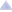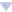### awoo's blog

By awoo, history, 12 days ago, translation,1837A - Grasshopper on a Line

Idea: BledDest

Tutorial
Solution (awoo)

1837B - Comparison String

Idea: BledDest

Tutorial
Solution (BledDest)

1837C - Best Binary String

Idea: BledDest

Tutorial
Solution (Neon)

1837D - Bracket Coloring

Idea: BledDest

Tutorial
Solution (BledDest)

1837E - Playoff Fixing

Idea: BledDest

Tutorial
Solution (awoo)

1837F - Editorial for Two

Idea: BledDest

Tutorial
Solution 1 (awoo)
Solution 2 (awoo)Comments (35)
 » another solution for F. my code
•  » » you can speed up the 'cal' function by using binary lifting
 » The solution for D looks a bit long. Here's mine
 » My solution for D, based on some observations.First of all check whether it is possible to make any coloring at all. This can be checked by just checking the balance of the whole string. If it's 0(basically equal number of '(' and ')') then coloring it is always possible, either as an RBS or as an reversed RBS or both. Only thing left to check is the number of colors. For this we can start pairing up the brackets(since there are equal number of brackets of both kind). We start by pairing the first '(' with the first ')' and color them based on a condition that if ')' comes before '(' then its reversed otherwise normal. This pairing will always result in either an RBS or an reversed RBS(try it out!).For example suppose we had colored this set: (()((())))and when pairing them according to this algorithm we had paired the first '(' with the first ')' even though for an RBS that is not the correct pairing. But still as a whole the string is an RBS!I haven't yet figured out a proof for this observation and will appreciate any and all inputs, but I found this a much simpler solution to code for D.
•  » » https://codeforces.com/contest/1837/submission/207216020Keep a running sum of the string: "(" is +1, ")" is -1. Mark anything positive as 1, mark anything negative as 2. It's that simple.
 » Is the D question simpler this time?
•  » » very much
 » $O(nlogn)$ is possible for F. We just iterate over all possible splits of array and try to greedily balance the sums between prefix and suffix. My solution
•  » » Could you pls explain why it's nlogn? It's not obvious to me that your inner "while" loop does constant (maybe amortized?) number of iterations.
•  » » » 10 days ago, # ^ | ← Rev. 3 →   Both while loops will be called at most $O(n)$ times:First one exchanges greatest element in multiset $ms$ with smallest one in $ms3$. Such moment that $ms3$ has smaller element than the greatest in $ms$ happens only whenever we expand prefix (which will only happen $O(n)$ times). It is quite obvious to see that there will be at most one such exchange after expansion of the prefix, so making it a while loop is quite redundant. Here's a accepted submission with an if statement instead of a while loop.Second while loop is easier to analyze since we may notice that it always increases $ms$ size by one and we are never decreasing it. Since $ms$ size is bounded by number of elements in input array, then again we get at most $O(n)$ calls.
 » 12 days ago, # | ← Rev. 2 →   In the tutorial for problem E, there is a statement that says:If both or neither of the teams are from 1 to 2^k, then the answer is immediately 0.However, it seems that the correct statement should be:If both or neither of the teams are from 1 to 2^(k-1), then the answer is immediately 0.or I didn't understand that part.
•  » » Thanks.I agree with your insight.
 » Problem F was qutie educational! I solved it with a binary search too, but instead of a bs over the answer, I used some segment trees to simulate like a frequency sort (using something similar to coordinate compression), then I binary seach on the amount of elements I take from the left $x$ and $k-x$ from the right, and similar to the editorial, the prefix sum from the left increase and the prefix sum from the right decrease, so we look for a balance (let those values be close as possible). Complexity: $O(n\log n \log n)$ (fast enough jeje) Solution
 » I managed to solve F using ternary search and binary search on segment tree. I had to make some hacky optimization to fit it in TL (3992ms)207420100
 » 11 days ago, # | ← Rev. 2 →   my solution for D:it is easy to check whether it is possible to make any coloring.if the answer is yes,check whether it is possible to coloring using 1 color;if it can't,the answer is 2.an easy way to coloring is:first,cut the string half from the middlecoloring all '(' in the left side using color 1.coloring all ')' in the right side using color 1.coloring all others using color 2.
•  » » Why does it work?
•  » » » if it is possible to make coloring,it can be showned that the counts of '(' on the leftside equals to the counts of ')' on the rightside.prove:consider that the counts of '(' on the leftside = a.the counts of '(' on the rightside = b.the counts of ')' on the leftside = c.the counts of ')' on the leftside = d.than a+b=c+d=n/2 (that is,the total count of '(' equals to the total count of')' )a+c=b+d=n/2 (that is,the total count of the leftside equals to the rightside.)so a=d&b=c.it means that the sequence of color 1:"(((())))" with (a) '(' and (d)')'the sequence of color 2:"))))((((" with (b) ')' and (c)'('.it's easy to showned both sequences is beautiful.
 » I think the tight limitation in problem F is unnecessary. My $O(n \log^2 n)$ solution got TLE on test 23.
 » Can someone please explain why 207472463 is getting TLE in java, but same logic in C++ works fine 207473463
•  » » The IO you are doing is slow; instead of Scanner, try BufferedReader,instead of System.out, Use PrintWriter.
 » 11 days ago, # | ← Rev. 2 →   In Problem F why the approach in which we remove maximum (n-k) elements and then minimizing the sum of k elements is not valid
•  » » We are finding some $min(max(a,b))$. Minimize $a+b$ does not necessary give the optimal answer. Take an example of $5 + 8 = 13$ and $7 + 7 = 14$, even though the sum is higher, we get a better result
•  » » » Thanks for the reply, can you provide a test case for that where it is not valid.
•  » » » » 1 4 3 2 3 2 4 Answer should be 4, but if you remove the max element the best you can do is 5.
•  » » » » » Thanks
 » For problem B, is it possible to create an array of 3 numbers for the following string >><>><>? I found that at least four numbers are needed. For example 5>4>3<4>3>2<3>2.
•  » » 3>2>1<3>2>1<3>2
 » Anyone please let help me find what is wrong with my solution of Comparison String problem. It has same idea. Here is my solution 207744443
•  » » The same mistake I made!You should find the longest continious increasing or decreasing sequenceThe problem with this idea that if you were increasing ,for instance 5 times, then decreasing 1, then increasing 5 again, you will count 1 to 5 then 4 then 5 to 9 which results in 10 but the answer should be that when decreasing you should begin counting again from 0 then the optimal answer be 6 not 10
 » Hey, can anyone tell me why my solution for question F is incorrect. I first found the k smallest element in o(nlogn ), then I put these in original order. I precalculated the sum of array and took a variable h=0; Then I iterated for n times the array , in which I did h+=a[i] and found minimum=min(minimun,max(h,s-h)); It should be done in o(nlogn). Here is my code
•  » » Greedy doesn't work. See above for a counter test case
 » Is C question was only on observation??
 » 8 days ago, # | ← Rev. 2 →   For problem F, I think there is a typo in the third last paragraph of tutorial. Index n should be used for head and n+1 should be used for tail.
 » 6 days ago, # |0.ComplaintFrame { display: inline-block; position: absolute; top: 0; right: -1.4em; } .ComplaintFrame a { text-decoration: none; color: #ff8c00; opacity: 0.5; } .ComplaintFrame a:hover { opacity: 1; } ._ComplaintFrame_popup p, ._ComplaintFrame_popup button { margin-top: 1rem; } ._ComplaintFrame_popup input[type=submit] { padding: 0.25rem 2rem; } ._ComplaintFrame_popup ul { margin-top: 1em !important; margin-bottom: 1em !important; } Why in problem E, the testcase: 3 -1 7 -1 8 2 -1 -1 5 has answer 4? Since team 2 and 4 are in positions 7 and 8 and 4 will be eliminated in the first round only.
 » 25 hours ago, # | ← Rev. 2 →   You can write F in $O(n~log^2~n)$ — 208816454Also the solution works fast (1590ms)